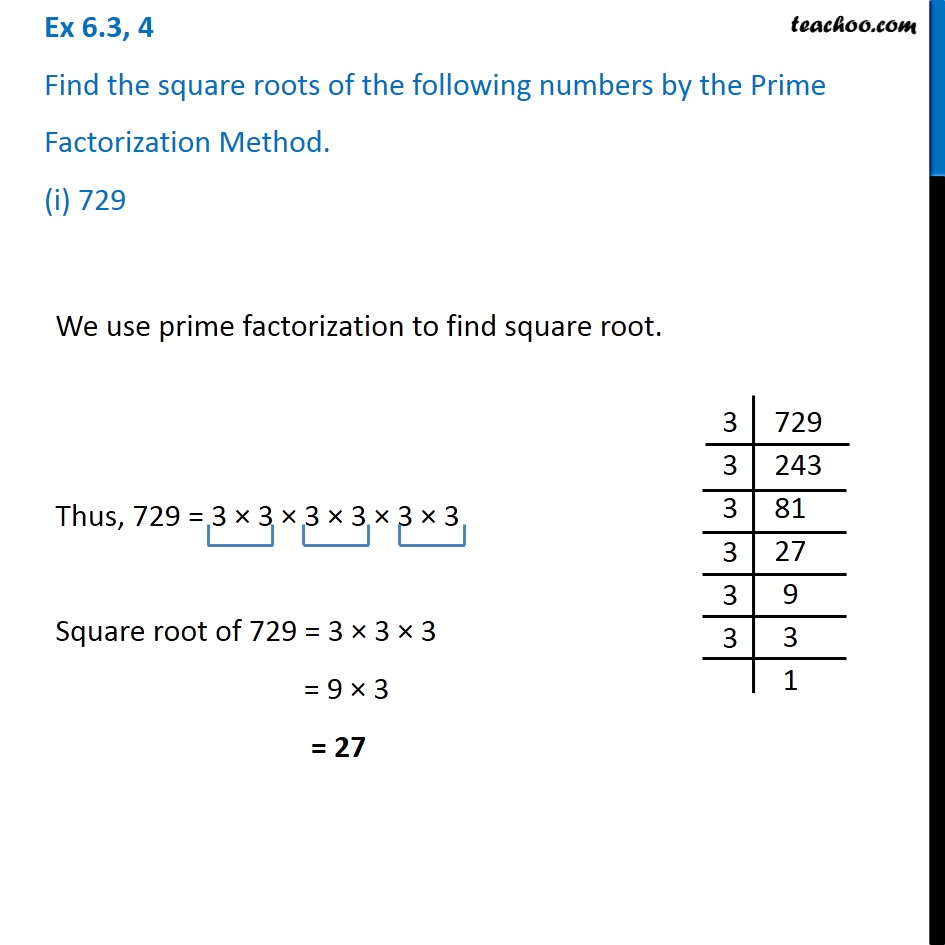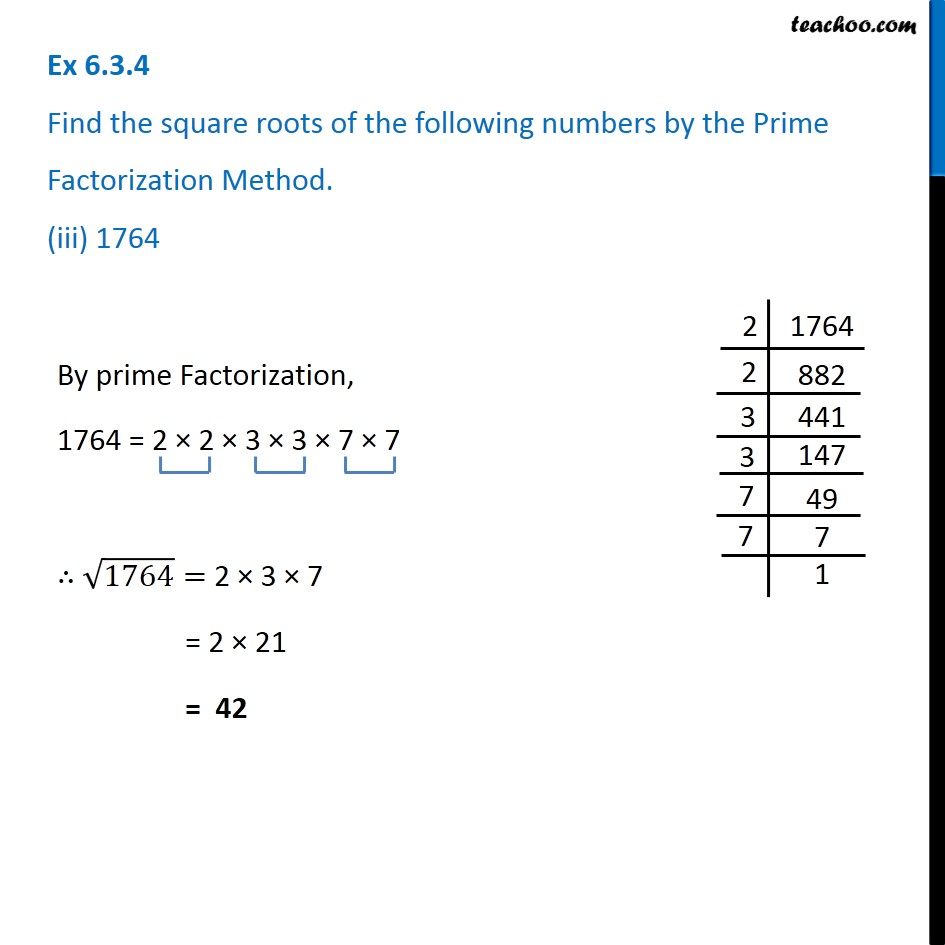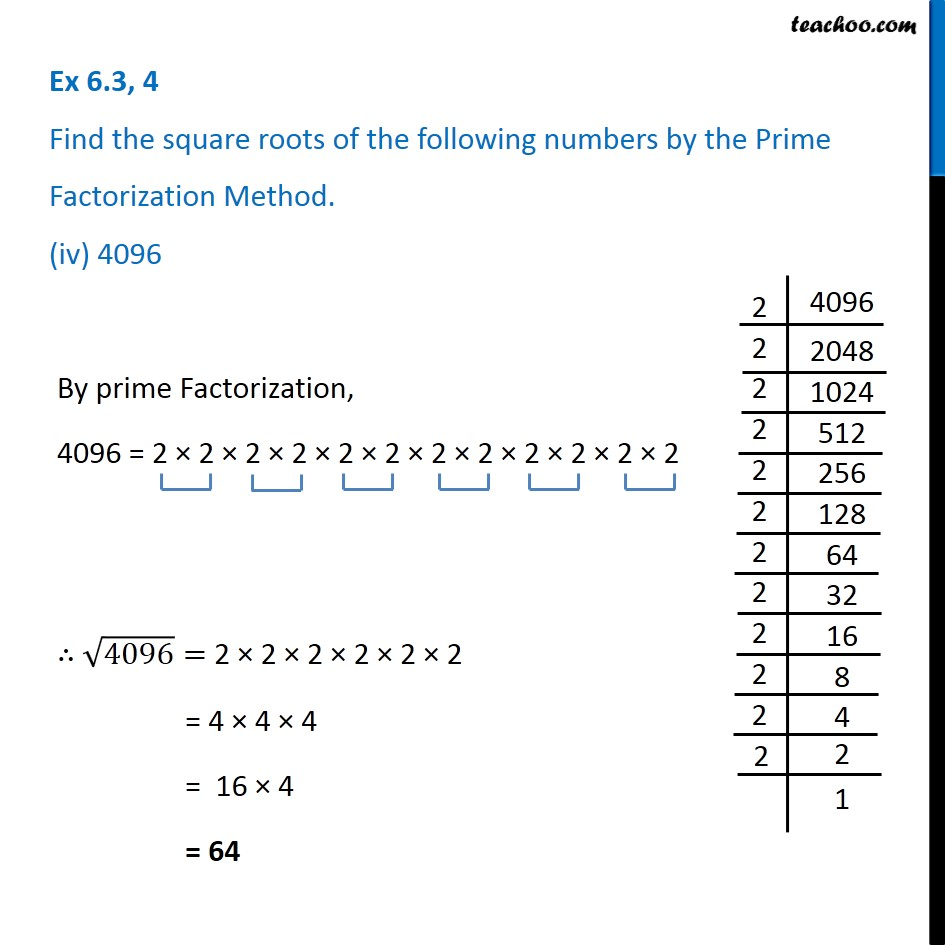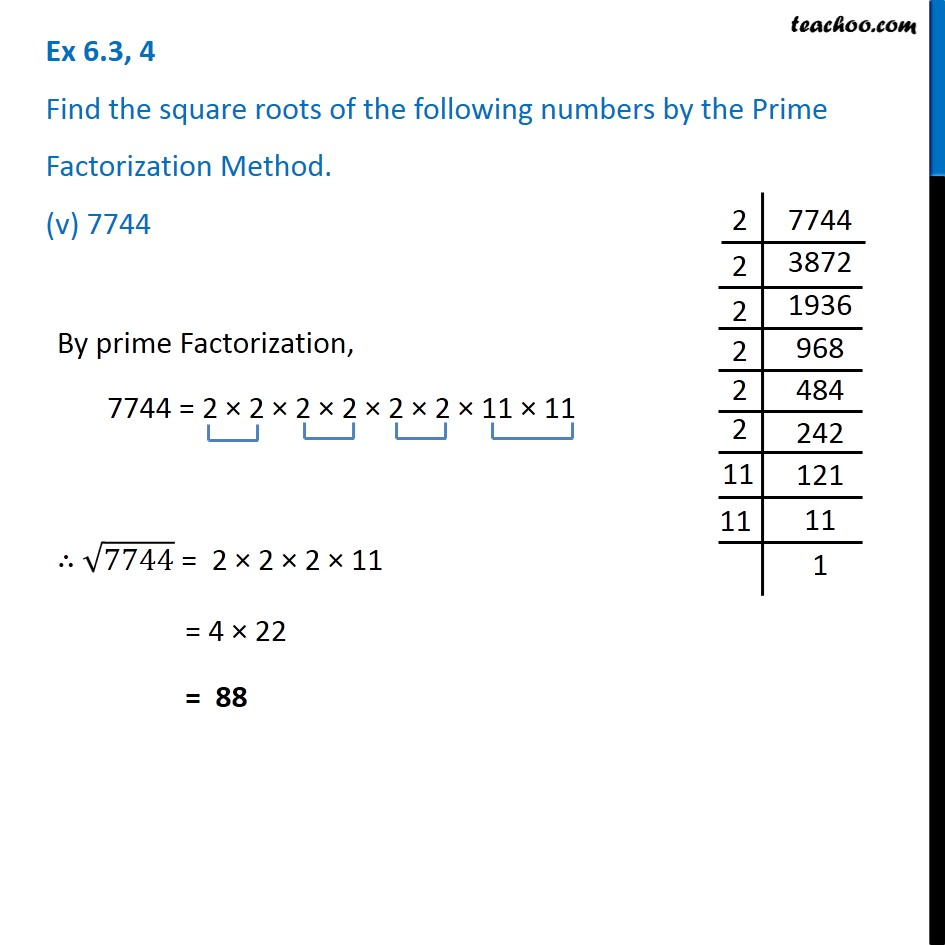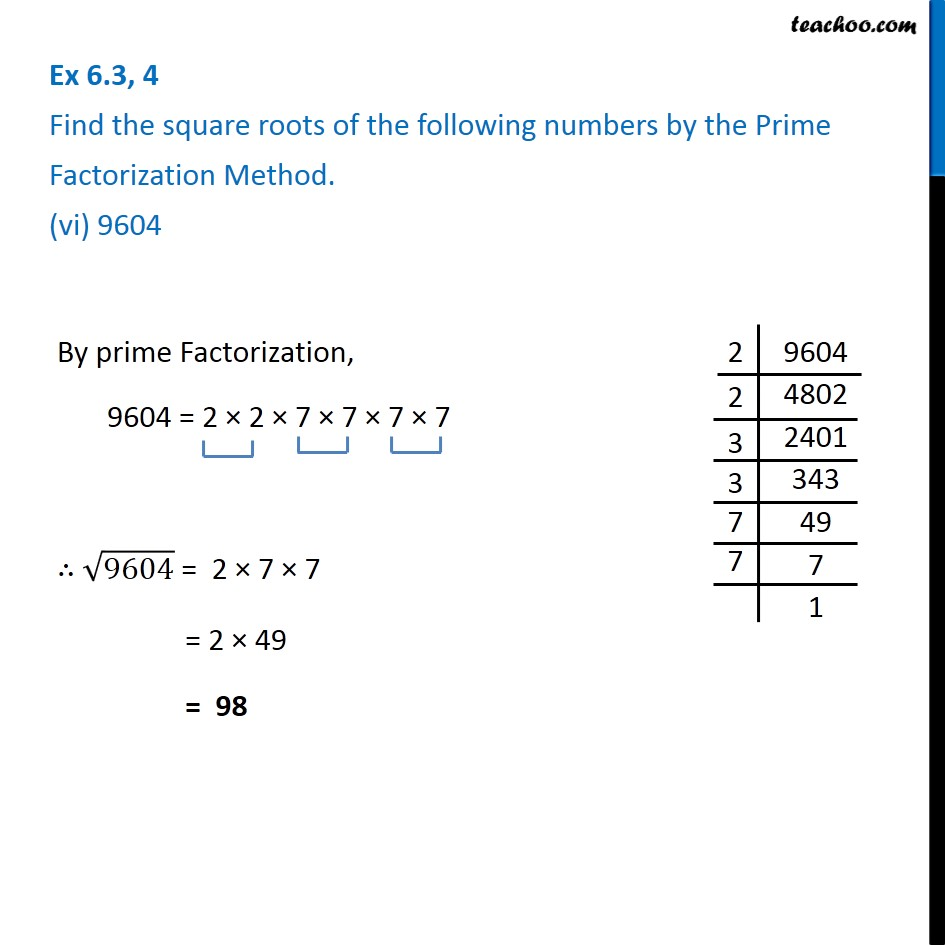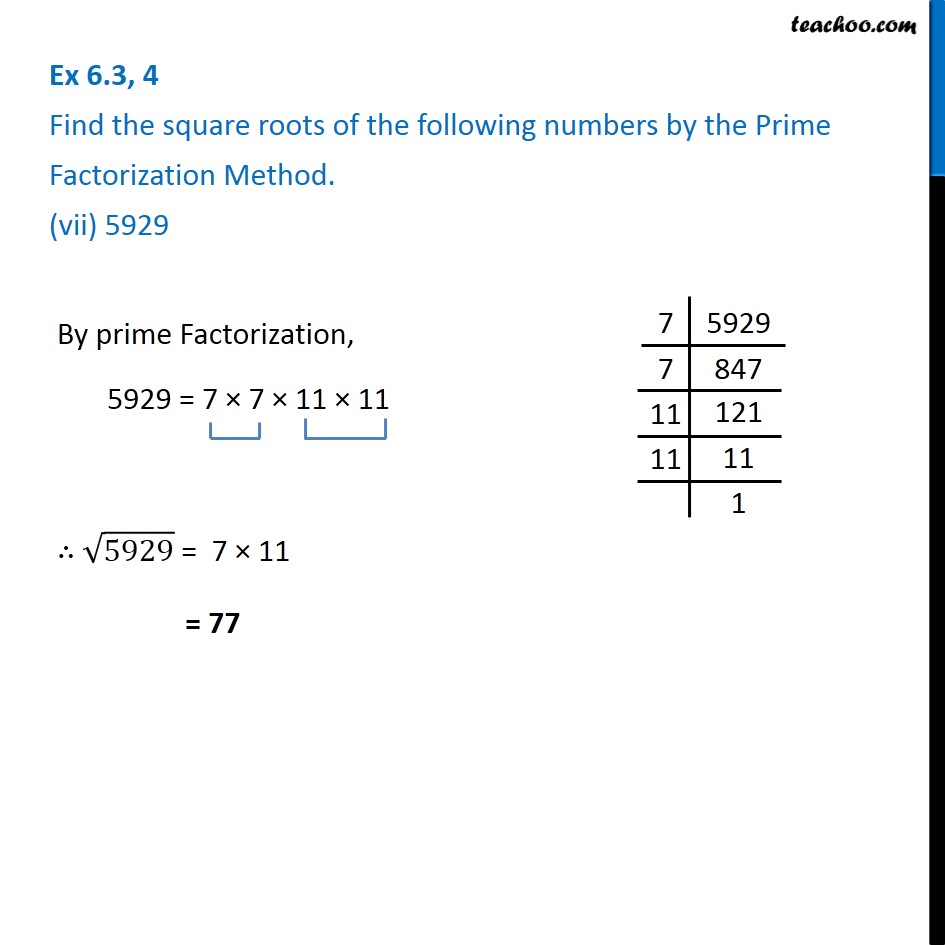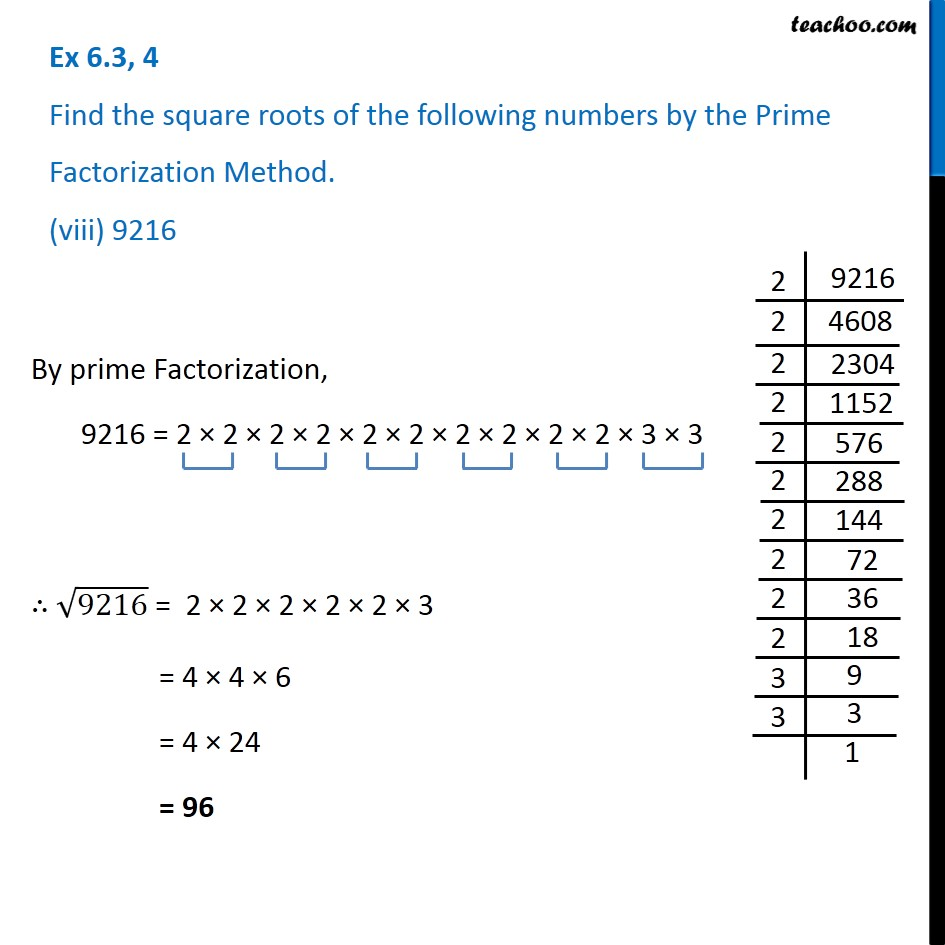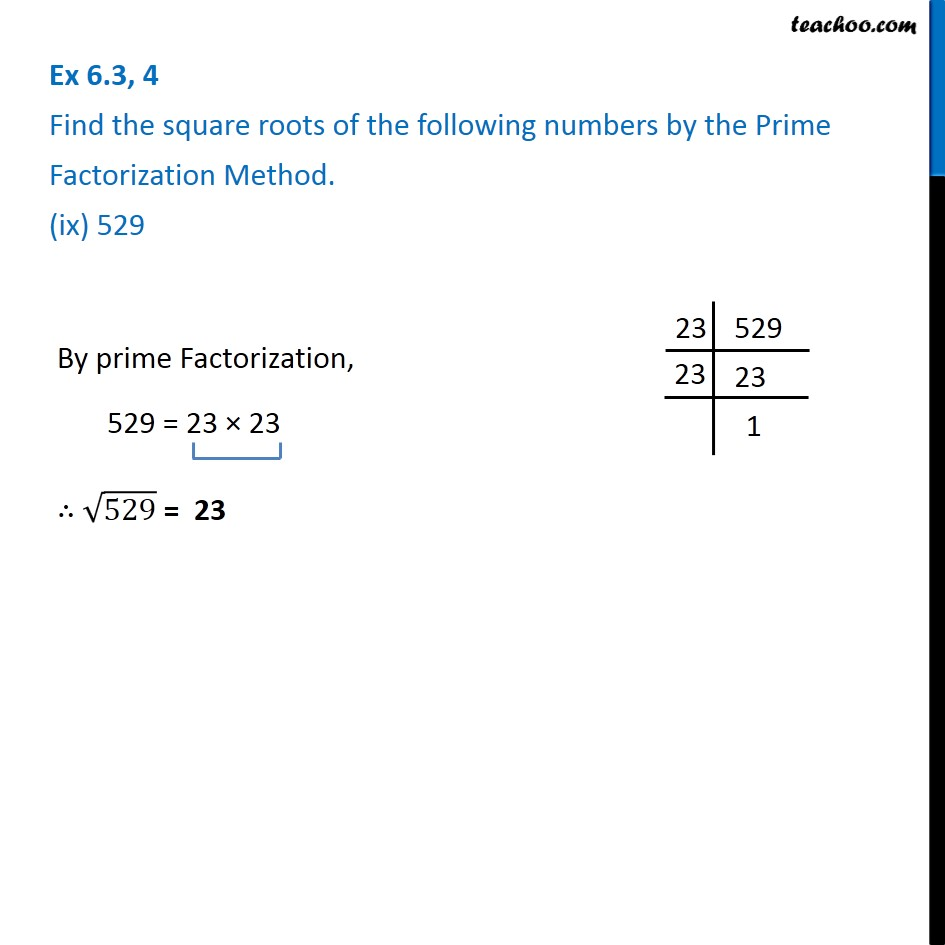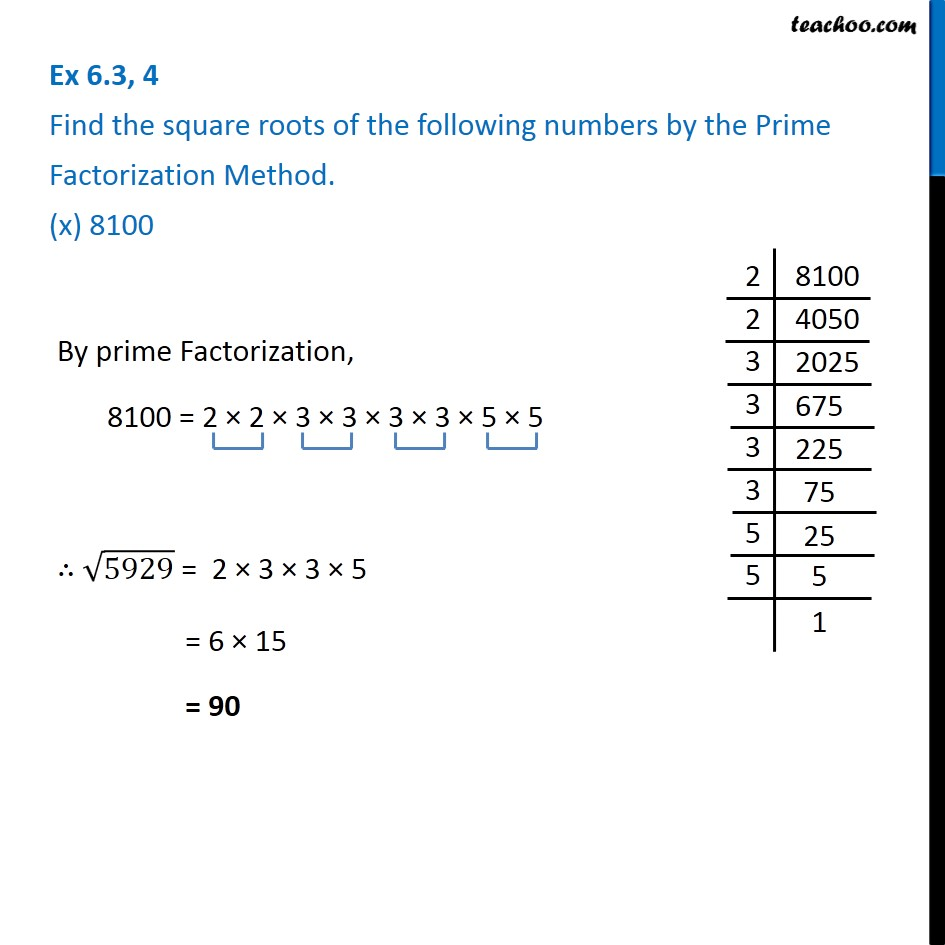X`

Subscribe to our Youtube Channel - https://you.tube/teachoo

1. Chapter 6 Class 8 Squares and Square Roots
2. Serial order wise
3. Ex 6.3

Transcript

Ex 6.3, 4 Find the square roots of the following numbers by the Prime Factorization Method. (i) 729We use prime factorization to find square root. Thus, 729 = 3 × 3 × 3 × 3 × 3 × 3 Square root of 729 = 3 × 3 × 3 = 9 × 3 = 27 Ex 6.3, 4 Find the square roots of the following numbers by the Prime Factorization Method. (ii) 400 We use prime factorization to find square root. Thus, 400 = 2 × 2 × 2 × 2 × 5 × 5 Square root of 400 = 2 × 2 × 5 = 4 × 5 = 20 Ex 6.3.4 Find the square roots of the following numbers by the Prime Factorization Method. (iii) 1764 By prime Factorization, 1764 = 2 × 2 × 3 × 3 × 7 × 7 ∴ √1764= 2 × 3 × 7 = 2 × 21 = 42 Ex 6.3, 4 Find the square roots of the following numbers by the Prime Factorization Method. (iv) 4096 By prime Factorization, 4096 = 2 × 2 × 2 × 2 × 2 × 2 × 2 × 2 × 2 × 2 × 2 × 2 ∴ √4096= 2 × 2 × 2 × 2 × 2 × 2 = 4 × 4 × 4 = 16 × 4 = 64 Ex 6.3, 4 Find the square roots of the following numbers by the Prime Factorization Method. (v) 7744 By prime Factorization, 7744 = 2 × 2 × 2 × 2 × 2 × 2 × 11 × 11 ∴ √7744 = 2 × 2 × 2 × 11 = 4 × 22 = 88 Ex 6.3, 4 Find the square roots of the following numbers by the Prime Factorization Method. (vi) 9604 By prime Factorization, 9604 = 2 × 2 × 7 × 7 × 7 × 7 ∴ √9604 = 2 × 7 × 7 = 2 × 49 = 98 Ex 6.3, 4 Find the square roots of the following numbers by the Prime Factorization Method. (vii) 5929 By prime Factorization, 5929 = 7 × 7 × 11 × 11 ∴ √5929 = 7 × 11 = 77 Ex 6.3, 4 Find the square roots of the following numbers by the Prime Factorization Method. (viii) 9216 By prime Factorization, 9216 = 2 × 2 × 2 × 2 × 2 × 2 × 2 × 2 × 2 × 2 × 3 × 3 ∴ √9216 = 2 × 2 × 2 × 2 × 2 × 3 = 4 × 4 × 6 = 4 × 24 = 96 Ex 6.3, 4 Find the square roots of the following numbers by the Prime Factorization Method. (ix) 529 By prime Factorization, 529 = 23 × 23 ∴ √529 = 23 Ex 6.3, 4 Find the square roots of the following numbers by the Prime Factorization Method. (x) 8100 By prime Factorization, 8100 = 2 × 2 × 3 × 3 × 3 × 3 × 5 × 5 ∴ √5929 = 2 × 3 × 3 × 5 = 6 × 15 = 90

Ex 6.3